# COOPERATION BY BINDING ELEMENTS

As we have seen earlier in this chapter, geographic databases are characterized by entities or classes which are organized through hierarchies whose common semantics are space inclusion. On the other hand, the model of MDDBs is mainly represented by the structure of its dimensions, hierarchies over dimensions, facts, and measures. MDDBs, besides the phenomenon of interest "what" and the temporal "when," support that of the spatial "where," often among several dimensions. Thus, the location dimension becomes the binding element between GDBs and MDDBs. The task of cooperation is based on two kinds of correspondences:

• Level correspondence maps a given geographic class of a geographic taxonomy to the corresponding level belonging to the location dimension and vice versa.

• Level instance correspondence results from the previous step and maps a generic instance of a geographic class to its corresponding instance of location dimension level and vice versa. In this step, for a correct correspondence, any instance changes should be propagated in both environments.

For a clear description of the above correspondences, we introduce some simple denotations. Let lg be a level of a location dimension whose domain is represented by dom(lg) and let Ig be its own instance. Let gc be a geographic class which belongs to the geographic taxonomy, and let the set of all its instances be represented by Ogc. Then, the above correspondences are achieved by a bijective function λ, where

• λ : gc lg for levels

• λ : Ogc dom(lg) for levels instances

The function λ, Full-Contains and Complete containment functions play a central role either in linking the heterogeneous environments or in providing data which lack in one environment by knowing data and their relationships in another. The following theorem shows how the location hierarchy of MDDBs can help to complete our partial knowledge about its equivalent hierarchy in GDBs.

Theorem 1: Let gc and gc be two geographic classes in a GDB such that gc Full-Contains gc. Let lg and lg be two geographic levels in an MDDB. If gc (gc) is mapped to lg (lg) by the function λ, then the complete containment function from lg to lg exists, and it is unique.

Proof. Let go and go be, respectively, an instance of gc and gc, such that between them the Full-Contains relationship exists. Applying the bijective function λ to these two instances, i.e., λ(go) = Ig and λ(go) = Ig, we obtain a generic pair <Ig, Ig>. The relationship between each pair of such geographic levels instances, obtained by λ onto the instances of gc Full-Contains gc, is a total and surjective function, i.e., it has the same properties as the complete containment function.

We prove that the complete containment function is unique and show the contrapositive.

Let the relationship <Ig, Ig> represent complete containment function. We assume there exists another complete containment function that maps another geographic level instance Ig (instead of Ig) to Ig. It indicates that the geographic instance corresponding to Ig, and obtained by the function λ1(Ig) = go is mapped to λ1(Ig) = go, and between them as well as between go and go Full-Contains relationships exist. It means that to different pairs < Ig, Ig>, < Ig,Ig> with different complete containment functions, different instances < go,go>, < go,go> with the same Full-Contain relationship correspond. This contradicts the Full-Contains definition.

Example 8: Let us consider two geographic classes MUNICIPALITY and PROVINCE in the GDB and let between them the Full-Contains relationship hold. Let us suppose only the geographic variable "Province" are defined in the MDDB. The geographic variable "Municipality" can be generated thanks to the above theorem by which the complete containment function is defined from "Province" to "Municipality."

Contrary to the above theorem and following the assumption made before about the equivalency of the geographic hierarchies, in both databases we can calculate the geometry of geographic objects. This is described in the following definition and example:

Definition 10: Let lg and lg be two geographic levels between which the complete containment function from lg to lg exists. Let gc be the geographic class corresponding to the geographic variable lg by λ. This mapping allows the generation of a geographic class gc such that gc Full-Contains gc. The geometric attribute value of any instance of the class gc = λ1(Ig) is obtained by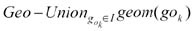where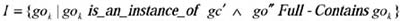Example 9: Let us suppose that in the MDDB two geographic variables "Municipality" and "District" are defined and that a complete containment function exists between them. We suppose that in the GDB only the geographic class "MUNICIPALITY" is defined. The mapping between the geographic class "MUNICIPALITY" and the geographic variable "Municipality" implies that for each instance of the variable "Municipality," one instance of the geographic class "MUNICIPALITY" exists and vice versa. In the GDB, the geographic class "DISTRICT" can be generated thanks to the DISTRICT Full-Contains MUNICIPALITY relationship. Since Full-Contains corresponds to the complete containment function, the "Geo-Union" of the geometric attributes of the class "MUNICIPALITY" instances will generate the geometric attribute of an instance of "DISTRICT."

### Definition of Binding Attributes

The actual cooperation is provided by linking dynamically the common geographic elements between GDBs and MDDBs. To achieve such a link, we extend the geographic class schema through some special attributes that describe all the phenomena of interests represented by cubes. For this reason, let us recall the definitions of geographic class schema and cube. According to this formulation, the set of attributes of geographic class can be subdivided into spatial and aspatial. The link that we mentioned before consists of adding new attributes, called binding attributes, to the set of aspatial attributes. A binding attribute of a geographic class is a cube derived from a given cube in a multidimensional database whose geographic level corresponds to the same class name. From now on, we will call such a cube a sub-cube. The binding attributes, in other words, represent all the phenomena (e.g., Car_Sales by model, month, municipality) modeled by cubes in MDDBs in the spatial context of geographic classes and objects.

The schema of a binding attribute is identical to the schema of the cube introduced in the previous section. However, the set of its levels is defined by all the levels of the cube except one, the geographic level, which is kept implicit by being equal to the class name (e.g., MUNICIPALITY). These are called local levels. Note that every binding attribute represents one and only one cube. For a clear description, we give the following example, and then we formalize the above concepts.

Example 10: Figure 3 shows graphically the binding attributes in the schema of the geographic class MUNICIPALITY.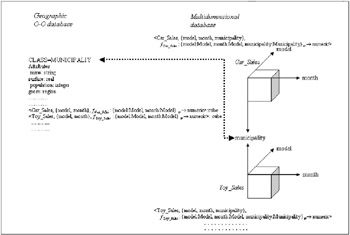Figure 3: Example of Binding Attributes in the Geographic Class Schema MUNICIPALITY

The following definition highlights the fact that the set of attributes in the schema of geographic class is enlarged by new binding attributes corresponding to cubes formed by only one location dimension.

Definition 11: Let C be a cube with the schema <Cname, L, fc : LT MT>, where L is formed solely by one geographic level lg. Let gc be a geographic class with the schema <n, sc, P, M>. If gc = λ1(lg), then the binding attribute AB of gc can be defined by the following schema <Cname, L lg, fc : {L lg}T M> and the set of attributes of gc is P AB.

The value of the binding attribute for an instance go of gc is defined by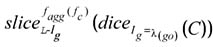, where Ig represents an instance of a geographic level lg.

Example 11: In Figure 4 the binding attributes of the instance (Rome) of the geographical class MUNICIPALITY refer to the corresponding instance (Rome) of the geographic level Municipality.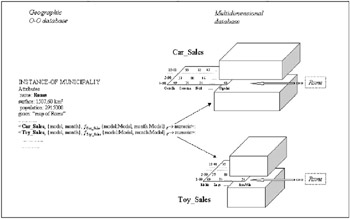Figure 4: Instances of the Binding Attributes, "Car_Sales" and "Toy_Sales"

Generally speaking, a cube can be defined with more geographic levels. The next definition describes the binding attributes when the number of geographic levels of the cube under consideration is equal or more than two.

Definition 12: Let the set of levels L of a given cube C = <Cname, L, fc : {L}T M> divided into geographic (Lg) and non-geographic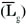levels. Let lg and lg be any two geographic levels belonging to Lg. Let gc and gc be two geographic classes, which are obtained by gc = λ1(lg) and gc = λ1(lg). The schemas of these last classes are, respectively, gc =< n, sc, P AB, M> and gc =< n, sc, P AB, M> where their binding attributes are defined as follows: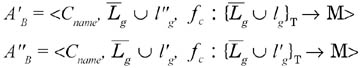The different geographic levels of a cube reflect the cases where each of them is a specialization of a given level of possibly the same location hierarchy. For instance, the level "Province of residence" is a specialization of the level "Province" in the location dimension hierarchy: Municipality Province Region. Consequently, its correspondence obtained by the function λ would also have been a specialization of the geographic class "PROVINCE" named "PROVINCE OF RESIDENCE."

While the process of the binding attributes generation for a "specialized" geographic class is the same as a "non-specialized" geographic class, the meaning of the attributes in the latter is intuitively more complex than those in the former. This is due to the specific organization of each geographic level along the relative dimension hierarchy, which will be discussed further through some illustrative examples.

Generally, in the case of cubes with more geographic levels, two different cases are distinguished as follows:

Case 1. There is no hierarchical relationship among the geographic levels of the cube.

Example 12: Let us consider a nationwide shoe company that owns chain stores located in all provinces. We assume that this company delivers monthly to its stores in various provinces a certain number of shoes from its production departments located in provinces. Then, the delivering of items is modeled by the following cube which has the "Province of Production," "Province of Distribution" as the geographic levels, and "month" as one non-geographic level.

` <Shoe_Sales,{Province of production, Province of distribution, Month},      fShoe_Sales : {Province of production : Province, Province of distribution : Province, month : Month}T → integer > `

As we can see, the above geographic levels are specializations of a single level "Province." In addition, the relative mapping by the function λ1 will yield two geographic classes with the same name which are sub-classes of the geographic class "PROVINCE."

` CLASS = PROVINCE OF PRODUCTION attributes name: string ..........     <Shoe_Sales,{Province of  distribution, month}, fShoe_Sales : {Province     of distribution: Province, month:Month}T >: cube and CLASS = PROVINCE OF DISTRIBUTION attributes name: string .............. <Shoe_Sales,{Province of    production, month}, fShoe_Sales :{Province of production: Province, month:Month}T >:cube `

The above example shows that in the geographic database, we have the generation of the geographic classes "PROVINCE OF PRODUCTION" and "PROVINCE OF DISTRIBUTION" which are specializations of the geographic class "PROVINCE."

We note the appearance of the geographic level "Province of distribution" (similarly Province of production) in the local levels of the binding attribute of the class "PROVINCE OF PRODUCTION" (similarly PROVINCE OF DISTRIBUTION).

Case 2. There is a hierarchical relationship among the geographic levels of the cube.

Example 13: Let us consider the same nationwide company of the above example that owns chain stores located in all provinces. This company delivers monthly a certain number of items to its stores from the production department located in various regions. It is represented by the following cube:

` <Shoe_Sales, {Province of distribution, Region of production, Month},        fShoe_Sales : {Province of production : Province, Region of distribution : Region, month : Month}T → integer >      CLASS = REGION OF PRODUCTION      attributes      name: string      ..............      <Shoe_Sales, {Province of distribution, month}, fShoe_Sales:{Province of      distribution: Province, month:Month}T >: cube      and      CLASS = PROVINCE OF DISTRIBUTION      attributes      name: string      ..............      < Shoe_Sales, {Region of production, month}, fShoe_Sales :{Region of      production: Region, month:Month}T >: cube `

The instances of the above geographic classes will assume different semantics. For example, a given instance of the first geographic class represents a region of shoe production in which the items are distributed over several provinces and months, while in the second case an instance represents the province of the distribution of shoes which are made in several regions and months.

### A Query Example

In this section, we show the solution of "Query 1" through the approach discussed above. Let us recall the query:

Query 1: "Find all the regions adjacent to the Tuscany region, in which the number of cars sold in 1990, for the <Corolla> product, was greater than 10,000."

The procedure for solving this query is formed by the following steps:

1. identification of the geographic class and geographic object/s involved by the geographic operator in the query;

In the case of our query, they are "Region" and "Tuscany" and the geographic operator is "Touching" (for a detailed description of this operator, see Ferri et al., 1999).

2. resolving the above Step 1;
The result of the geographic operator Geo-Touching is formed by the instances: "Liguria, Emilia-Romagna, Umbria, and Latium."

3. identification of the binding attribute in the geographic class which is the "target" of the query;
In our case, "Car_Sales" in "regions."

4. selection of the cube identified by the binding attribute in the MDDB; In our query, select the cube "Car_Sales."

5. satisfying the constraints on the non-geographic dimension of the cube;
In our case according to the specific predicates, dice operator is applied on the values of Time and Model dimensions.

6. satisfying the constraints on the measure;
In our query, it will involve all the instances of the measure.

7. selection of the values of the measure which satisfy the previous constraints; In our query, they are the regions with the number "greater than 10,000" of "Corolla" cars sold in "1990."

8. Pass the list of the geographic variable instances that satisfy the query to the GDB. This means identifying the corresponding geographic objects.

In the extended geographic environment, the above query may be expressed by the following SQL-like expression:

` SELECT  R1.name FROM    REGION R1,R2 WHERE   R1.Car_Sales(model=Corolla,   year=1990)>10,000 AND   R1.geom Geo-Touching R2.geom AND   R2.name=Tuscany `

Let us recall that the binding attribute is obtained from the formula as follows: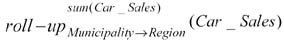where the containment function Municipality Region is the concatenation of the two containment functions Province Region and Municipality Province. This means that the above formula is equivalent to the application of the following double roll-up operator on "Car_Sales":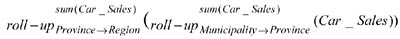To summarize, the query is solved through the following steps:

• in the GDB:

`    Region Geo-Touching Region="Tuscany" `
• and in the MDDB: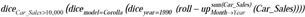Note that all the geographic operations are performed entirely in the GDB, the results of which are automatically reported in the MDDB in order to apply OLAP operators. Thanks to the equivalence properties between these environments, the so-called OLAP results are indicated as the extended part of the geographic objects involved in the query.Multidimensional Databases: Problems and Solutions
ISBN: 1591400538
EAN: 2147483647
Year: 2003
Pages: 150